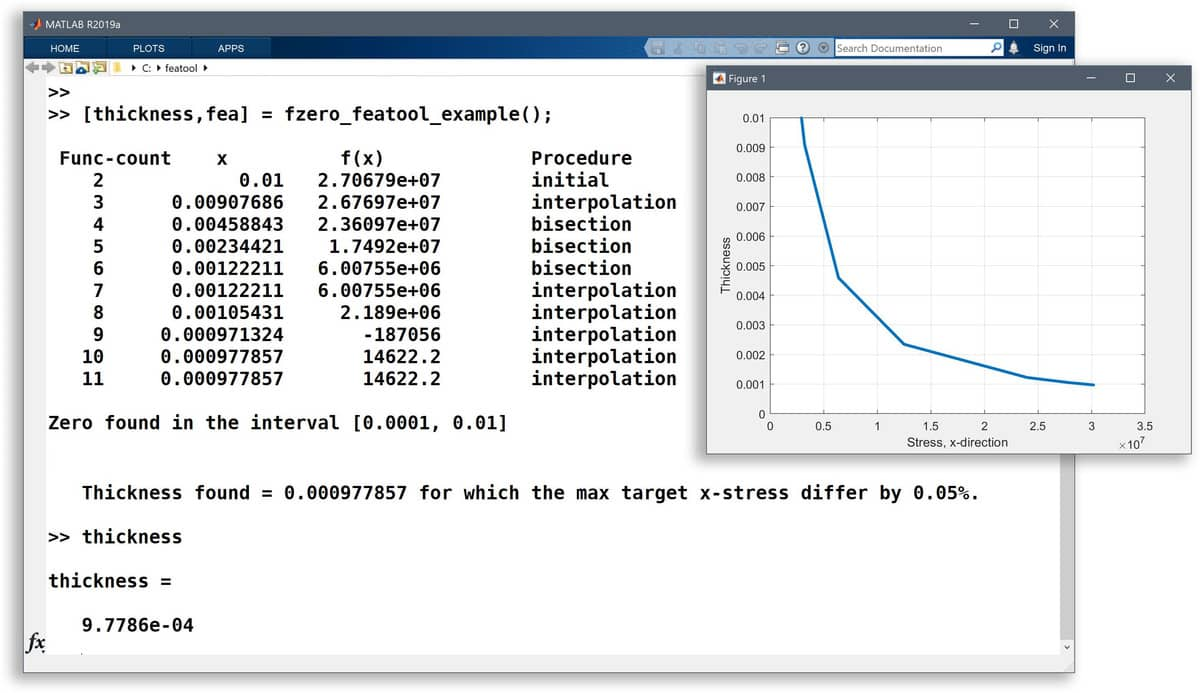# Inverse Modeling and Parameter Search Using the fzero MATLAB functionFEATool Multiphysics not only features an easy to use graphical user interface (GUI), but is also fully compatible with the MATLAB scripting language. Models can both be saved in binary format and MATLAB m-script files (every action in the GUI is recorded and has a one to one corresponding FEATool MATLAB function call). This also means that FEATool FEA simulation models can be used with all built-in MATLAB functionality as well as external toolboxes like the Optimization Toolbox and Simulink.

The following post illustrates how to use the built-in MATLAB function fzero to conduct a quick and simple inverse parameter search. The hole in plate FEATool quickstart and benchmark model, is used as a starting point, and instead of finding an unknown the stress for a given thickness, fzero is used to find for what unknown thickness a given prescribed stress is found. This could be useful in finding a critical material limit and other design constraints.

The basic process is to define a MATLAB function that takes a one parameter argument, in this case the plate thickness, and return the difference from the sought value (here the stress in the x-direction). The problem function is in this example defined as an anonymous MATLAB function, but could just as well be implemented as a regular function.

``````pfun = @(thickness) sx_target - compute_max_stressx(thickness);
``````

This function is called by fzero with appropriate arguments

``````result_thickness = fzero( pfun, thickness_range );
``````

The stress computation function can looks something like the following

``````function [ sx_max ] = compute_max_stressx( thickness )

fea = get_fea_struct( thickness );   % Finite element fea problem struct.

fea.sol.u = solvestat( fea, 'fid', [] );   % Call solver without terminal output.

% Define an expression for the stress in the x-direction.
E11  = 'E_pss/(1-nu_pss^2)';
E12  = ['nu_pss*',E11];
s_sx = [E11,'*ux + ',E12,'*vy'];

[~,sx_max] = minmaxsubd( s_sx, fea );   % Calculate the maximum stress in the x-direction.
``````

where `get_fea_struct` assembles and returns a FEATool finite element struct, as in the example ex_planestress1 file.

Running this script we find that fzero takes 11 tries in the search to successfully narrows down the thickness to 0.001, which yields an error of about 0.05%.